Test: Electrostatics

# Test: Electrostatics - Electrical Engineering (EE)

Test Description

## 20 Questions MCQ Test GATE Electrical Engineering (EE) 2023 Mock Test Series - Test: Electrostatics

Test: Electrostatics for Electrical Engineering (EE) 2023 is part of GATE Electrical Engineering (EE) 2023 Mock Test Series preparation. The Test: Electrostatics questions and answers have been prepared according to the Electrical Engineering (EE) exam syllabus.The Test: Electrostatics MCQs are made for Electrical Engineering (EE) 2023 Exam. Find important definitions, questions, notes, meanings, examples, exercises, MCQs and online tests for Test: Electrostatics below.
Solutions of Test: Electrostatics questions in English are available as part of our GATE Electrical Engineering (EE) 2023 Mock Test Series for Electrical Engineering (EE) & Test: Electrostatics solutions in Hindi for GATE Electrical Engineering (EE) 2023 Mock Test Series course. Download more important topics, notes, lectures and mock test series for Electrical Engineering (EE) Exam by signing up for free. Attempt Test: Electrostatics | 20 questions in 60 minutes | Mock test for Electrical Engineering (EE) preparation | Free important questions MCQ to study GATE Electrical Engineering (EE) 2023 Mock Test Series for Electrical Engineering (EE) Exam | Download free PDF with solutions
 1 Crore+ students have signed up on EduRev. Have you?
Test: Electrostatics - Question 1

### A spherical surface of radius of 3 mm is centered at P(4, 1, 5) in free space. If D = xux C/m2 the net electric flux leaving the spherical surface is

Detailed Solution for Test: Electrostatics - Question 1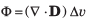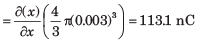Test: Electrostatics - Question 2

### The electric flux density is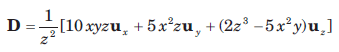The volume charge density ρv at (-2, 3, 5) is

Detailed Solution for Test: Electrostatics - Question 2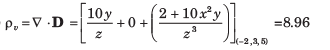Test: Electrostatics - Question 3

### If D = 2rur C/m2, the total electric flux leaving the surface of the cube, 0 < x , y, z < 0.4 is

Detailed Solution for Test: Electrostatics - Question 3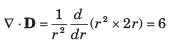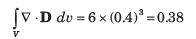Test: Electrostatics - Question 4

E = 4ux -3 uy + 5uin the neighborhood of point P(6, 2,  -3). The incremental work done in moving 5 C charge a distance of 2 m in the direction ux + uy + uz is

Detailed Solution for Test: Electrostatics - Question 4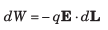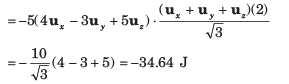Test: Electrostatics - Question 5

If E = 100up  V /m , the incremental amount of work done in moving a 60 μC charge a distance of 2 mm from P(1, 2, 3) toward Q(2, 1, 4) is

Detailed Solution for Test: Electrostatics - Question 5

The vector in this direction is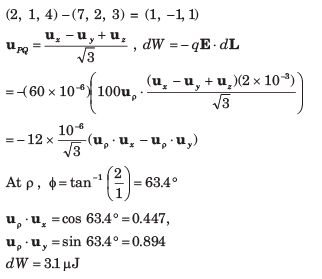Test: Electrostatics - Question 6

A 10 C charge is moved from the origin to P(3, 1, -1) in the field E = 2xux - 3y2 uy + 4uz V/m along the straight line path x = -3y, y = x + 2z. The amount of energy required is

Detailed Solution for Test: Electrostatics - Question 6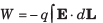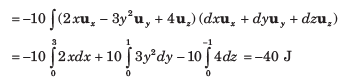Test: Electrostatics - Question 7

A uniform surface charge density of 30 nC /m2 is present on the spherical surface r = 6 mm in free space. The VAB between A (r = 2 cm, θ = 350 , Ø = 550 ) and B (r = 3 cm, θ = 400 , Ø = 900 )

Detailed Solution for Test: Electrostatics - Question 7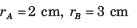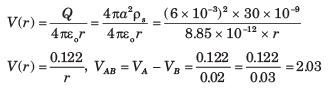Test: Electrostatics - Question 8

A point charge is located at the origin in free space. The work done in carrying a charge 10 C from point A (r = 4 cm, θ = π/6 , Ø  = π/4) to B (r = 4 cm, θ = π/3 , Ø  = π/6) is

Detailed Solution for Test: Electrostatics - Question 8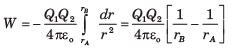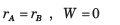Test: Electrostatics - Question 9

Let a uniform surface charge density of 5 nC/m2 be present at the z = 0 plane, a uniform line charge density of 8 nC/m be located at x = 0, z = 4 and a point charge of 2 μC be present at P(2, 0, 0). If V = 0 at A(0, 0, 5), the V at B(1, 2, 3) is

Detailed Solution for Test: Electrostatics - Question 9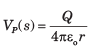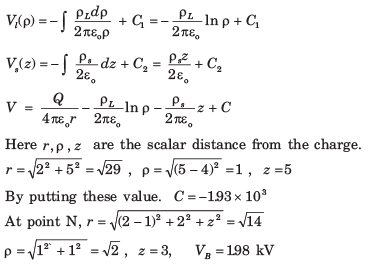Test: Electrostatics - Question 10

A non uniform linear charge density, ρL = 6/ (z2 + 1) nC/m lies along the z axis. The potential at P(ρ = 1, 0, 0) in free space is (V = 0 )

Detailed Solution for Test: Electrostatics - Question 10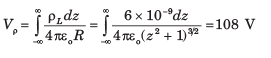Test: Electrostatics - Question 11

The annular surface, 1 cm  < ρ < 3 cm carries the nonuniform surface charge density ρs = 5ρ nC/m2. The V at P(0, 0, 2 cm) is

Detailed Solution for Test: Electrostatics - Question 11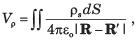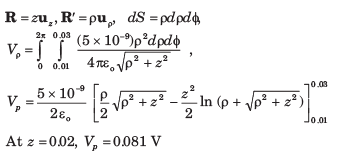Test: Electrostatics - Question 12

If V = 2xy2z3 + 3ln(x2 + 2y2 + 3z2 ) in free space the magnitude of electric field E at P (3, 2, -1) is

Detailed Solution for Test: Electrostatics - Question 12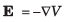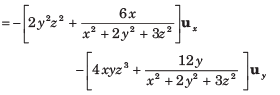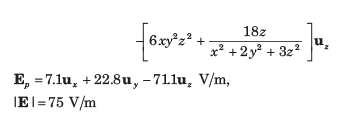Test: Electrostatics - Question 13

It is known that the potential is given by V = 70 r0.6 V. The volume charge density at r = 0.6 m is

Detailed Solution for Test: Electrostatics - Question 13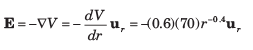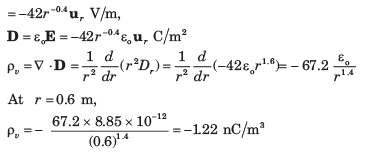Test: Electrostatics - Question 14

The potential field V = 80r2 cosθ V. The volume charge density at point P(2.5, θ = 300 , Ø = 600 ) in free space is

Detailed Solution for Test: Electrostatics - Question 14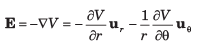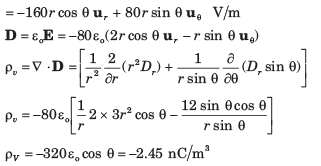Test: Electrostatics - Question 15

Within the cylinder ρ = 2, 0 <  z  <1 the potential is given by V = 100 + 50ρ +150ρ sinØ V. The charge lies within the cylinder is

Detailed Solution for Test: Electrostatics - Question 15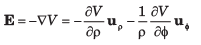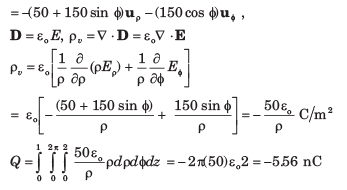Test: Electrostatics - Question 16

A dipole having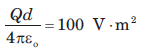is located at the origin in free space and aligned so that its moment is in the uz direction. The E at point (r = 1, 450  ,Ø = 0) is

Detailed Solution for Test: Electrostatics - Question 16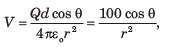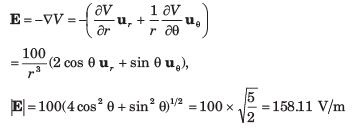Test: Electrostatics - Question 17

A dipole located at the origin in free space has a moment p = 2 x 10-9 uz c.m. The points at which |E|θ  = 1 mV m on line y = z , x = 0 are

Detailed Solution for Test: Electrostatics - Question 17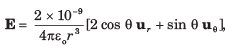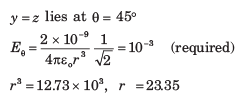Test: Electrostatics - Question 18

A dipole having a moment p = 3ux - 5uy + 10uz  nC.m is located at P(1, 2,-4) in free space. The V at Q (2, 3, 4) is

Detailed Solution for Test: Electrostatics - Question 18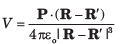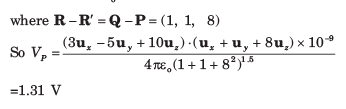Test: Electrostatics - Question 19

A potential field in free space is expressed as V =40/xyz . The total energy stored within the cube 1 <  x, y, z < 2 is

Detailed Solution for Test: Electrostatics - Question 19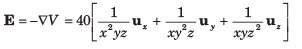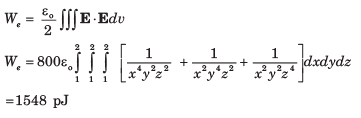Test: Electrostatics - Question 20

Four 1.2 nC point charge are located in free space at the corners of a square 4 cm on a side. The total potential energy stored is

Detailed Solution for Test: Electrostatics - Question 20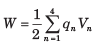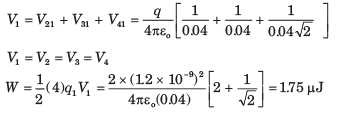## GATE Electrical Engineering (EE) 2023 Mock Test Series

22 docs|284 tests(Scan QR code)